# Engineering Mathematics - Online Test

Q1. A box contains 4 white balls and 3 red balls. In succession, two balls are randomly selected and removed from the box. Given that the first removed ball is white, the probability that the second removed ball is red is
Explaination / Solution:
No Explaination.

Q2. An Eigenvector of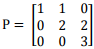is
Explaination / Solution:
No Explaination.

Q3. For the differential equation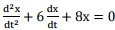with initial conditions x(0) = 1 and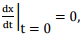the solution is
Explaination / Solution:
No Explaination.

Q4. For the set of equations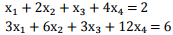The following statement is true
Explaination / Solution:
No Explaination.

Q5. The trace and determinant of a 2 × 2 matrix are known to be –2 and –35 respectively. It’s Eigenvalues are
Explaination / Solution:
No Explaination.

Q6. If E denotes expectation, the variance of a random variable X is given by
Explaination / Solution:

The variance of a random variable x is given by E X − E X

Q7. The following plot shows a function which varies linearly with x . The value of the integral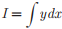isExplaination / Solution:

The given plot is straight line whose equation isQ8. For |x| << 1, coth (x) can be approximated as
Explaination / Solution: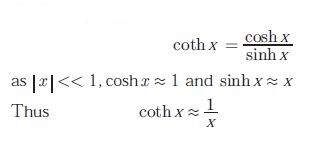Q9.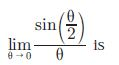Explaination / Solution: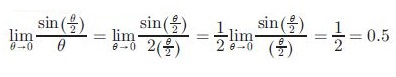Q10. Which one of following functions is strictly bounded?
Explaination / Solution: